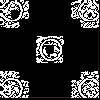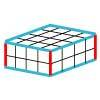#### You may also like### Doplication

We can arrange dots in a similar way to the 5 on a dice and they usually sit quite well into a rectangular shape. How many altogether in this 3 by 5? What happens for other sizes?### Round and Round the Circle

What happens if you join every second point on this circle? How about every third point? Try with different steps and see if you can predict what will happen.### Making Cuboids

Let's say you can only use two different lengths - 2 units and 4 units. Using just these 2 lengths as the edges how many different cuboids can you make?

# Always, Sometimes or Never? Number

##### Age 7 to 11Challenge Level

Are the following statements always true, sometimes true or never true?
How do you know?

Can you find examples or counter-examples for each one?

For the 'sometimes' cards can you explain when they are true? Or rewrite them so that they are always true or never true?

 The sum of three numbers is odd If you add 1 to an odd number you get an even number Multiples of 5 end in a 5 If you add two odd numbers you get an odd number If you add a multiple of 10 to a multiple of 5 the answer is a multiple of 5

What about these more complex statements?

 When you multiply two numbers you will always get a bigger number If you add a number to 5 your answer will be bigger than 5 A square number has an even number of factors The sum of three consecutive numbers is divisible by 3 Dividing a whole number by a half makes it twice as big

You could cut out the statement cards and arrange them in this grid.

Alternatively, you may like to try out your ideas using the interactivities below: